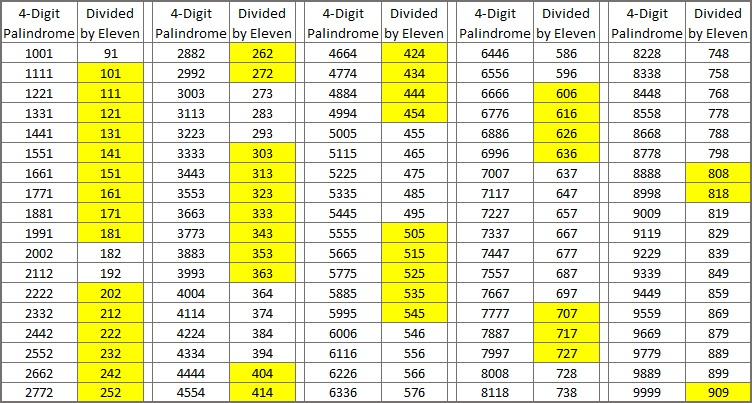# 1661 A Little About Palindromes

Contents

### Today’s Puzzle:

A number is a palindrome if it reads the same backward as it does forward. For example, 1661 is a 4-digit palindrome. Today’s puzzle asks you to explore number palindromes and their factors.Half of the time when a 4-digit palindrome is divided by 11, we get a 3-digit palindrome. Why does that happen?Are there 3-digit palindromes that were NOT included in the Divided by Eleven column in the table above?

All 2-digit palindromes are divisible by 11. They are 11, 22, 33, 44, 55, 66, 77, 88, 99.

There are only eight 3-digit palindromes that are divisible by 11. They are 121, 242, 363, 484, 616, 737, 858, 979. Some 3-digit palindromes are prime numbers. Others are divisible by 101 or 111. Still, there are plenty of 3-digit palindromes that are composite numbers but not divisible by 11, 101, or 111.

Most 5-digit palindromes are NOT divisible by 11. I was able to construct one that is, 76967, because the red digits minus the blue digits are 2312 = 11, a number divisible by 11.

Here’s another: 81818. It works because 242 = 22, a number divisible by 11.

What 5-digit palindrome can you construct that is divisible by 11?

Will an N-digit palindrome be divisible by 11? What difference does it make if N is an even number or if N is an odd number?

### Factors of 1661:

1661 is divisible by 11 because the red digits minus the blue digits equal 0, a number divisible by 11.

• 1661 is a composite number.
• Prime factorization: 1661 = 11 × 151.
• 1661 has no exponents greater than 1 in its prime factorization, so √1661 cannot be simplified.
• The exponents in the prime factorization are 1 and 1. Adding one to each exponent and multiplying we get (1 + 1)(1 + 1) = 2 × 2 = 4. Therefore 1661 has exactly 4 factors.
• The factors of 1661 are outlined with their factor pair partners in the graphic below.### More about the Number 1661:

1661 is also a palindrome in base 18:
1661₁₀ = 525₁₈ because
5(18²) + 2(18¹) + 5(18º) =
5(324) + 2(18) + 5(1) =
1620 + 36 + 5 =
1661.

This site uses Akismet to reduce spam. Learn how your comment data is processed.